# Grade - math word problems

#### Number of examples found: 5328

• Today in schoolThere are 9 girls and 11 boys in the class today. What is the probability that Suzan will go to the board today?
• Volume of ballFind the volume of a volleyball that has a radius of 4 1/2 decimeters. Use 22/7 for π
• Cage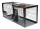How many m2 of mesh farmer use for fencing of a cuboid cage with dimensions 25m, 18m, and 2.5m?
• Tailor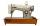Tailor bought 2 3/4 meters of textile and paid 638 CZK. Determine the price per 1 m of the textile.
• Circle from string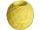Martin has a long 628 mm string . He makes circle from it. Calculate the radius of the circle.
• Base, percents, valueBase is 344084 which is 100 %. How many percent is 384177?
• 5 pumps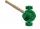Five pump to pump 3 hours 1600 hl of water. How long pumped same amount of water 4 pumps?
• RhombusPQRS is a rhombus. Given that PQ=3 cm & height of rhombus is given 2 cm. Calculate its area.
• Simple interest 3Find the simple interest if 11928 USD at 2% for 10 weeks.
• Decimal expansion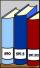Calculate: 2 . 1 + 0 . 10 + 7 . 10000 + 4 . 1000 + 6 . 100 + 0 . 100000 =
• The product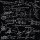The product of a number plus that number and its inverse is two and one-half. What is the inverse of this numberThe fruit basket is five times heavier than the empty basket. Filled is 20kg heavier than empty. Determine the weight of the fruit in the basket.
• Distance between 2 pointsFind the distance between the points (7, -9), (-1, -9)
• Degrees 2The temperature was 3°F and falls four degrees Fahrenheit. What is actually temperature?
• Reciprocal equation 2Solve this equation: x + 5/x - 6 = 4/11
• VariableFind variable P: PP plus P x P plus P = 160
• Type of triangle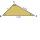How do I find the triangle type if the angle ratio is 2:3:7 ?
• Percents - easyHow many percent is 432 out of 434?
• Dividing by five and tenNumber 5040 divide by the number 5 and by number 10: a = 5040: 5 b = 5040: 10
• Parametric equationFind the parametric equation of a line with y-intercept (0,-4) and a slope of -2.

Do you have an interesting mathematical word problem that you can't solve it? Submit a math problem, and we can try to solve it.

We will send a solution to your e-mail address. Solved examples are also published here. Please enter the e-mail correctly and check whether you don't have a full mailbox.

Please do not submit problems from current active competitions such as Mathematical Olympiad, correspondence seminars etc...